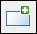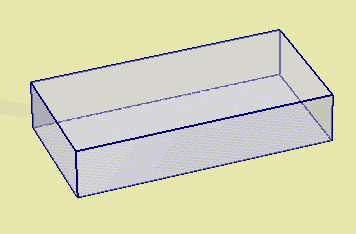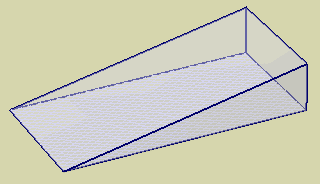Expert Modelling: Hands-on help from Rocscience Engineers. Register Here

## Search Results

A rectangular or square load can be defined with the Add Rectangular Load option. The load magnitude can be uniform or variable, and the load application can be staged.

1. Select Add Rectangular Loadfrom the toolbar or the Loads menu.
2. You will see the Define Load dialog for rectangular loads. Enter the following information:
• Rectangle Properties (length, width, angle)
• Load Properties (magnitude, direction, depth / bottom elevation, staging information, load type)
• Display Properties (optional)
3. See below for details about the input parameters. When you are finished entering the load information, select OK.

4. You will now be prompted to define the location of the rectangular load in the Plan View. The location of a rectangular load is defined by entering the x,y coordinates of the center of the rectangle. This can be entered graphically with the mouse, by clicking at the desired location in the Plan View. Or you can use the keyboard to type in the x,y coordinates in the prompt line.
5. As soon as the load location has been entered, the load will be added to the model, and displayed in both the Plan View and 3D View.The parameters which define a rectangular load are described below.

## Rectangle Properties

Length and Width

The size of a rectangular load is defined by entering Length and Width.

Angle

The orientation of a rectangular load is defined by entering the Angle with respect to the X-axis of the Plan View. The Angle is defined as the counter-clockwise angle between the X-axis and the Length dimension of the rectangle.

NOTE: a rectangular load cannot be converted into a general polygon using the load editing options. If you require a more complex load shape, then use the Add Polygonal Load option to define the load.

For a rectangular load, you may choose Load Type = Flexible or Rigid.

Flexible

For a Flexible load you can define the load magnitude as a uniform or variable Pressure. A Flexible load implies that the stress distribution on the ground immediately beneath the load is equal to the loading stress distribution.

Rigid

For a Rigid Load, you can define the load magnitude as a uniform or variable Pressure, or as a Force and two Moments. A Rigid load implies that the displacement immediately beneath the load remains planar. The stress distribution immediately beneath the load is not (in general) equal to the loading stress distribution, due to the rigid displacement constraint. For rigid loads, it is recommended to use multi-layered stress computation method.

NOTE: rigid loads cannot be used with time-dependent consolidation analysis in Settle3, only with immediate elastic settlement or long term consolidation.

See the Settle3 Theory Manual for further information.

If the Boussinesq or Westergaard stress computation methods are selected, and the load type is defined as Flexible, then the following load direction options are available:

• Vertical
• Horizontal
• Vertical and Horizontal

If any of the other stress computation methods are selected, or the load type is set to Rigid, only vertical loads can be defined.

Load magnitude is defined in terms of force / area (i.e. distributed load or pressure). The pressure can be uniform or variable. For rigid loads you also have the option of defining the load magnitude as a Force and two Moments.

Uniform Pressure

To define a uniform load magnitude, enter the pressure (force per unit area) in the Pressure edit box. This will define a load with a uniform magnitude over the area of the rectangle.

Variable Pressure

To define a load magnitude which varies over the area of the rectangle, select the Variable checkbox and select the Define button. You will see a dialog which allows you to define the load magnitude at each corner of the rectangle.

NOTE: the load magnitude at each corner is defined as a distributed load (i.e. force / area). You are NOT defining point loads, you are defining the magnitude of the distributed load, at each corner. It is up to the user to determine the appropriate magnitude at each point, which correctly models the desired load distribution.

The load distribution over the area of the rectangle is obtained by interpolation using the load magnitudes at the four corners. For example, a load which varies from zero along one edge of the rectangle, to a maximum value at the opposite edge, might appear as follows in the 3D View.

Variable load magnitude over a rectangular areaForce and Moments

For Rigid loads you can define the load magnitude as a single vertical Force and two Moments (Mx and My) with x and y axes. The force is applied through the centroid of the load. If you apply moments, the settlement under the load will not be horizontal, but will follow an inclined planar function.

If your model uses multiple stages, the load can be applied at any stage, or applied in increments at different stages, as described in the Load Staging section below.

In defining a horizontal flexible load, the same options exist as for a flexible vertical load - a uniform or variable pressure can be defined, and the load can be staged. The Angle from X axis can also be defined for a horizontal load. The Angle is defined as the counter-clockwise angle between the X-axis and the direction of the horizontal load.

A load can be applied on the ground surface or at any depth below the ground surface.

Horizontal Soil Layers

If the Horizontal Soil Layers option is selected in the Soil Profiles tab of the Project Settings:

• If the vertical axis is defined as Depth below Ground Surface, the load depth is defined by Depth.
• If the vertical axis is defined as Elevation with a Ground Elevation specified, the load depth is defined by Bottom Elevation. The default elevation is that specified in the Project Settings.

Non-Horizontal Soil Layers

If the Non-Horizontal Soil Layers option is selected in the Project Settings, and the Non-Horizontal Ground Surface option is NOT selected, then the load depth / elevation is defined in the same way as when the Horizontal Soil Layers option is selected.

If the Non-Horizontal Soil Layers and the Non-Horizontal Ground Surface options are selected, the load depth is defined by Bottom Elevation. The default elevation is that specified in the Project Settings.

If your model uses multiple stages, the depth of the load can be staged, as described below.

NOTE: if you apply a load at some depth below the ground surface, the soil above the load depth is NOT assumed to be excavated, it is assumed that the soil layers remain in place. If you wish to define loading within an excavation, then you must use the Add Excavation option to define an excavation, and then define the loading. See the Add Excavation topic for details.

If your model uses multiple stages, the load can be applied at any stage, or applied in increments at different stages using the Advanced Staging option.

Installation Stage

If you wish to apply a load at a particular stage of a multi-stage model, then use the Installation Stage option to select the desired Installation Stage.

1. Select the Advanced Staging checkbox. The dialog will expand, allowing you to enter a Load Factor and Depth for each stage.
2. The Load Factor is a multiplying factor for the Load Magnitude. For example:
• If you want to double the load magnitude at a given stage, enter Load Factor = 2 for that stage.
• If the load does not exist at a given stage, enter Load Factor = 0.
• If you want the original load magnitude, enter Load Factor = 1.
• NOTE: if the Load Magnitude is Variable (see above), then the Load Magnitude at each vertex of the load will be multiplied by the Load Factor.
3. The Depth can be staged by entering the Depth of the load application at each stage. The actual depth from ground surface is entered, NOT a factor. The depth is entered as a positive number. If you are modeling an excavation, see the note above regarding Load Depth and the Add Excavation option.

If your load is staged, it is a good idea to select the Stage Tabs, after adding the load, to check that the load is applied at the correct stage(s), and that the magnitudes and depths are correct. If not, then use the Edit Load Properties option, and make sure that the correct values have been applied at the correct stages.

## Display Properties

The appearance of a load can be customized by selecting the Display Properties button in the Define Load dialog. You will see the Load Display Properties dialog, in which you can define:

• Label - this is an optional text label / name, which can be used to identify the load, and will appear in the Info Viewer listing, right sidebar View Controls, and Data Tips.
• Outline / Fill / Hatch - customize the colours / hatch pattern used to display the load.
• Height - the load Height option allows you to define a constant height for the load. This is for display purposes only, and has no effect on the load magnitude or analysis. If you do not define the load Height, an apparent load height is automatically determined based on the load magnitude.

NOTE: Load Display Properties can be customized for each individual load. Load Display Properties can also be accessed by right-clicking on a load, and selecting Display Properties from the popup menu.